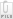Pay results fee

Sorry, you have not enough credits to pay results fee.

# semi-algebra/topology

Launcher Dr. Terry Allen WA
Status Vaulted: Apr 17, 2012 18:29

### Labels

Algebraic topology Affine geometry

### Description

This completes my topology paper: 1. Import 3 files for appendix 2. Post on line so I can edit article 3. Make changes as needed to finalize paper. 4. Write a forwarding letter to publisher describing your contribution and opinion of the paper. Per our agreement you may bill hourly if the work exceeds the estimate.appedix 2.docxMusic is the Finite Union of Notes and Intervals .pdfAppendix III Mathematic Theorems Used in This Study.docx

### Zomb Results

Results for this zomb have been placed in the vault.

You can access it for free.

Music is A Clock, Not a Donut: Cipher Theory of Dual Tonality By Dr. Terry Allen tallen@wildblue.net

April 17, 2012

Abstract:

A defined musical system must have at least 2 functions f(x) and g(x) forming a composite function with at most 1 center (Music Multicentricity Theorem).

Thus tonality is the line of projection of the composite function f x g x h where f(x) is a+b+c = 0, g(x) > 0 is a scale center, and h(x) .> 0 is an instrument center. These are semi-algebraic function describes by van den Dries.

As defined here: A music cipher is an affine projection that marries R:Z pitch:position to compose a note (tone point = (pitch value, position value)) by a vector that embeds a harmonic image in a musical system, or makes a new note from one already known in a system, only by cipher vector additions to a barycenter according to a difference function.

The cipher vector is the complete lattice of the musical system, precisely the vector between sub lattices,and this cipher vector defines the Boolean Arithmetic Operator of the Music Cipher and also the geometry of tone value spaces formed by its prime ideals.

For example, open strings on guitar are the prime ideals of the tuning, the chords and scales are the tone value space of the tuning. Therefore the observe musical key is not the guitar key.

Music is composed of a topology where the binary union of notes and open intervals (defined by the composite functions of the fundamental, the key, and the intonation algorithm), so I assert here that tonality (totality of expression) is precisely the cipher vector formed by the projection between the logic sub lattice and and at least 2 algebraic sub lattices of the musical system, where the sub lattices all share the fundamental as 1 common center.

Therefore the cipher is a line (between fundamental and key) and a point ("the key") at once, resulting from line-point duality in geometry.

Without an R : Z cipher the musical clock is incomplete, not simple, and undefined: Pitch Value Space (the torus) without a cipher is therefore not a valid concept. Music cannot possibly have 2 equal centers. Euler's donut ("the tonnetz") is dead.

Note that the fundamental tone is not a pitch value nor a string value but a union of the 2 tone values as (f(x), g(x)) = (pitch, position)

The new model is a clock: the fundamental is the hour hand, the in- strument position is the minute hand, and scale position is the second hand.

Tonality, like time on the clock, is a vector composite of 3 functions with 1 fundamental in common. Therefore, tonality has at least 2 functions but at most 1 center.

Hence I define a myriad of Musical Abelian pairs f x g that are cryptologically orthogonal tone value functions: (pitch, position), (string, fret), (tuning vector, summation vector), (redundancy, playability), (harmony, melody), (syntax, semantics), (algebra, logic), (algorithm, cipher), (originality, expressivity), which I assert all reflects the fact that tone value space (not pitch value space) is a Hilbert Space.

Finally we have the pair (tonality, harmony). This is a novel idea.

First I describe musical arithmetic using the concept of a cipher vector, a difference function between 2 dysjunct tone values sets forming the line of tonal projection that marries pitch to position.

After I show the orthogonal algebra and logic of the cipher in Hilbert Space, and the geometry of the musical lattice, I grow the musical topos as a triplet M = (x;{f(x)}; {g(x)}), developing semi-algebraic theorems in Euclidian fashion as I go to explain the origin of the Abelian pairs I observed.

This leads to the anomalous conclusion that music is a clock defined by at least 2 hands connected to at most 1 center. Pitch Value Space without an R : Z cipher algorithm to encode the position values in Z is undefined.

After almost 300 years Euler's Donut is killed by a Guitar Player!Dr Allen Write Up.pdf
Comments for results

No comments yet.

Disclaimer

Zombal does not in anyway guarantee the quality or correctness of the vaulted results. The results have been compiled by members on the site and have not been reviewed by a third party.

However, we encourage members to comment on vaulted work to improve its accuracy. If you're able to provide suggestions for improvement and to point out errors, you may be presented with the opportunity for paid follow-up work.